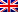#[Sharif University CTF 2016] [Crypto 100 – Universal re-encryption] Write Up

## Description

Universal Re-Encryption
Let p be a prime, and g be an element of ℤ/pℤ of prime order q.
Let x ∈ ℤ/qℤ be the private key, and h = g^x (mod p) be the public key.

To encrypt a message m ∈ ℤ/pℤ, pick two random values r, s ∈ ℤ/qℤ, and compute the ciphertext as follows:
(a, b, c, d) = (g^r, h^r, g^s, mh^s).

Download a valid ciphertext σ = (a, b, c, d) below, and compute another valid ciphertext σ′ = (a′, b′, c′, d′) such that:
σ and σ′ decrypt to the same message;
a ≠ a′ and b ≠ b′ and c ≠ c′ and d ≠ d′.

## Resolution

This challenge was based on an application of the property of an hypothetic homeomorphic encryption which is that we can operate on the cipher as we would do on the clear text (without decoding it before).
So basically we must re-encrypt the ciphertext in order to make decryption giving the same result on both ciphers.

We found a very useful link which explains this ciphering method : https://crypto.stanford.edu/~pgolle/papers/univrenc.pdf.

Then we applied what is described at page 6 with this python code :

```p = int('0x8000048d1d71b57838b7d90ebc63b8c853f3af1af87ce2db5593f3386ae5139d040d3844e31db723d39cdd7717c8cffc26f6f877b5c85ca8e595ca687c07c773',16)

b = int('0x436c161645052a76c1f7c976da63f61987f5f9bf7cb810a0e6fb1ea593aa9397c7b7cb0488f0f14cf93c79eef967a4b2a39388da1a357077d30a6f8b2a2c97e7',16)
d = int('0x1a50308011b409460d504cc7cddd61cdff1bda0774d1329b59606df274bce81a7e4b15830ddd4e684e3f2422d36bd52220134881db560be0a34c76a9c5bbb6be',16)

r = 5
s = 7

print hex((pow(a,s,p))%p)
print hex((pow(b,s,p))%p)
print hex((c*pow(a,r,p))%p)
print hex((d*pow(b,r,p))%p)
```

Flag was : “SharifCTF{d03de378ab0f370b0a27e7e366ba6f2e}“.